# Multi-part exercises

## Multi-part questions

Multi-part questions require more than one calculation to solve them. Most story problems are multi-part questions. Such questions require strategic thinking to decompose the problem into subproblems and combine the partial results.

Consider the story problem below. We will learn how to create this question using the Arithmetic Notebook question type.

Example problem

Amy wants a subscription for two years. She is in doubt about whether she should go for option A or option B.

How much cheaper is option A than option B for a two-year subscription?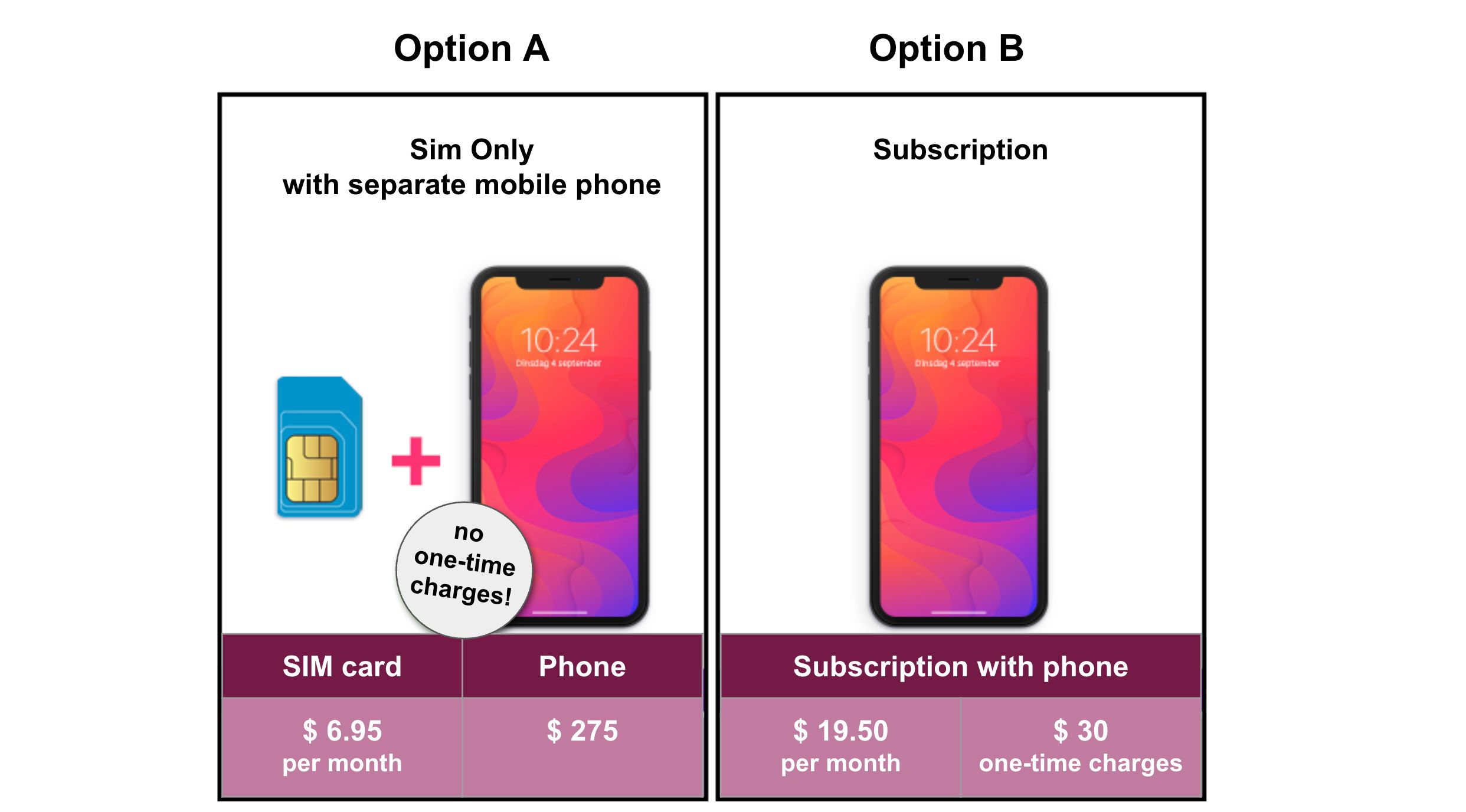## Solution models

The table below shows a solution model for our example problem.

Part Description Calculation Marks
$N_{months}$ The number of months $2\times 12$ 0
$C_A$ The total costs of option A $N_{months}\times 6.95 + 275$ 1
$C_B$ The total costs of option B $N_{months}\times 19.50 + 30$ 1
$solution$ How much option A is cheaper than option B $C_B-C_A$ 1

If you are a teacher or have designed assessment questions before, you probably are familiar with such solution models.

Solution models have the following properties:

• Every part represents something, such as "The total costs for option A." Algebrakit uses this to generate helpful hints and error feedback, as we will see later.
• Some parts depend on other parts. For example, to calculate $C_B$, you must first calculate $N_{months}$. These dependencies reveal what strategies are suitable to solve the problem.
• You can assign marks to each part to allow for partial scoring.
• You do not have to specify the part solutions. Algebrakit handles this automatically.

## Adding the solution model to Arithmetic Notebook

The image below shows how you define this solution model in Arithmetic Notebook: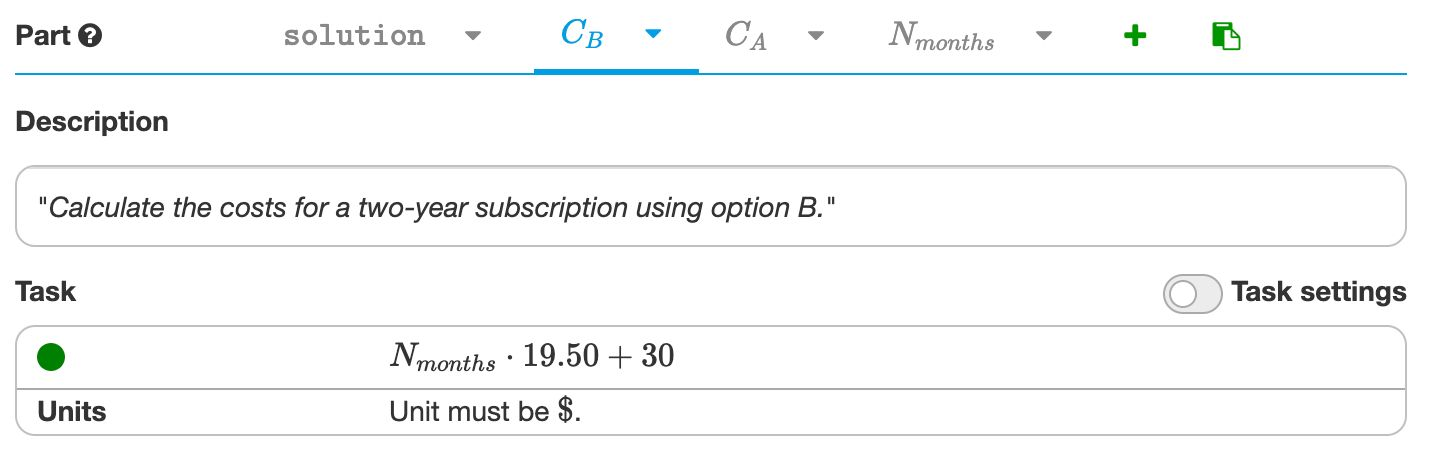Figure: The solution model in Arithmetic Notebook. Click on the Edit button of the live example to see this screen in your browser.

As shown in the figure, the Arithmetic Notebook question allows you to add multiple parts. Each part has a name, a description, and a task.

• You add a new part by clicking the + button at the top right.
• You can change the part name through the dropdown menu next to it.
• The part description consists of a verb and a definition. In this example, the verb is 'Calculate,' and the definition is 'the costs for a two-year subscription using option B.' Algebrakit sometimes uses only the definition and sometimes the definition and verb.

The live example below shows the result after we created the Arithmetic Notebook question. Go ahead and solve the problem step by step. Or click the Edit button to see how we made it.

Live example: The arithmetic story problem we are creating.

## Multiple strategies

What if there are multiple valid strategies to solve a problem? For example, you can find the answer to our example problem as follows:

Part Description Calculation Marks
$N_{months}$ Number of months $2\times 12$ 0
$d_{monthly}$ How much is option A cheaper per month than option B $19.50-6.95$ 0.5
$D_{monthly}$ The difference of the total monthly costs between options A and B $N_{months}\times d_{monthly}$ 0.5
$D_{fixed}$ The difference of fixed costs between options A and B $275-30$ 1
$solution$ How much option A is cheaper than option B $D_{monthly} - D_{fixed}$ 1

You can see that this second strategy introduces new parts. The $solution$ part does exist in both approaches, although the definitions differ.

You can add this second strategy to your Arithmetic Notebook question by adding the new parts. In the solution step, you add the second calculation by hovering over the taskbar and clicking the + button.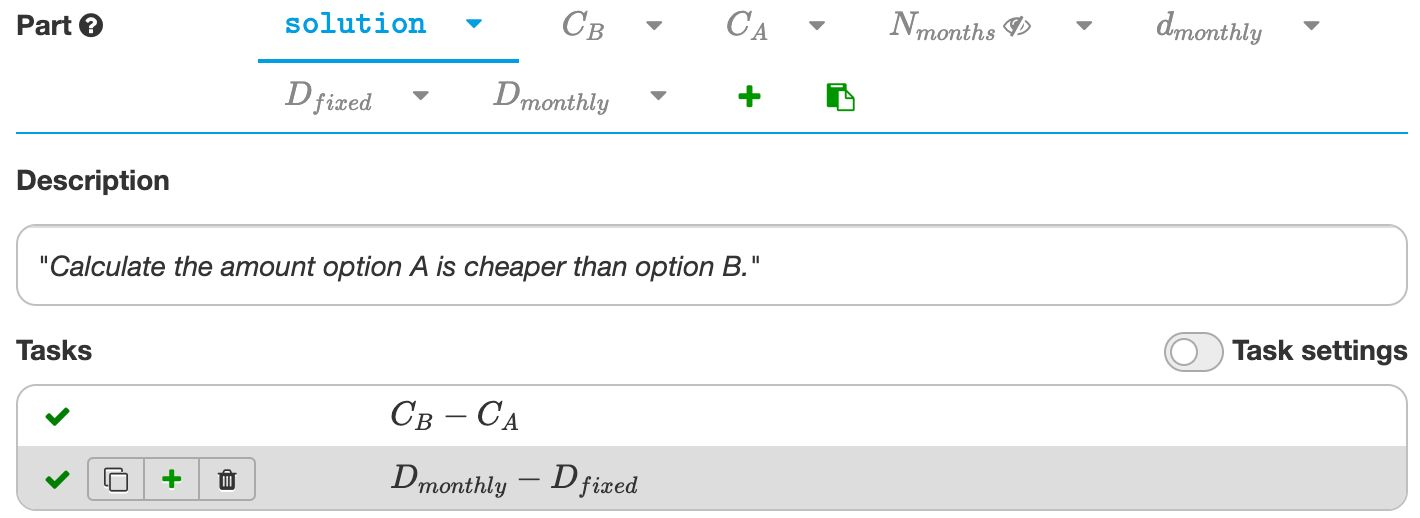Figure: Adding a second strategy to the solution model in Arithmetic Notebook.

## How Algebrakit uses the solution model

Algebrakit uses the solution model to generate worked solutions, provide personalized support, and support partial marking.

### Generate structured solutions

Algebrakit uses the solution model to generate a worked solution with a clear structure. Algebrakit also shows the description of every input that was matched to the solution model. This helps students connect their mathematical work to the concepts they are calculating.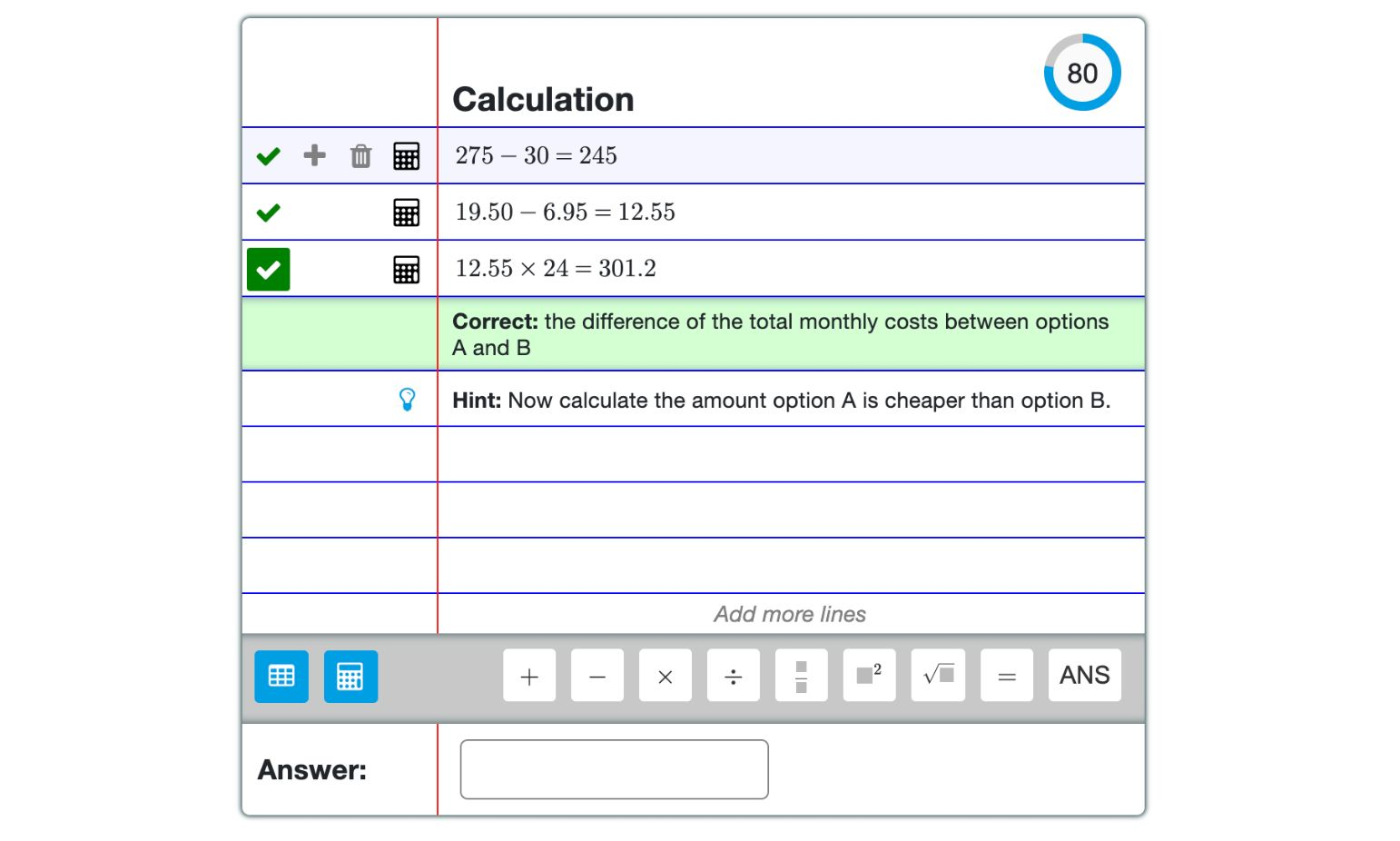Figure: Algebrakit adds descriptions to correct inputs.

### Personalized support following the student's strategy

If a solution model has multiple solution strategies, as in our example problem, Algebrakit will track the student's strategy. In the example below, the hint depends on what parts the student was working on.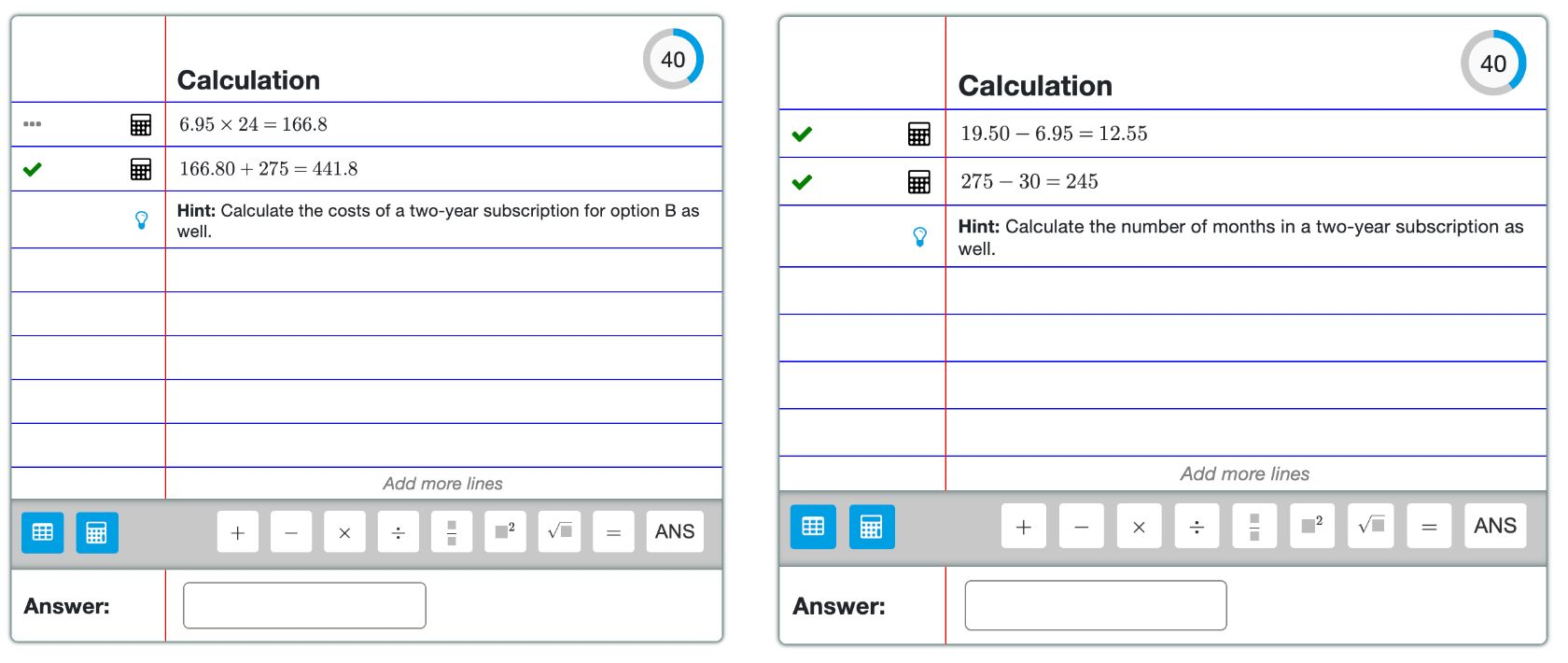Figure: Left and right show partial solutions from both strategies. Algebrakit shows a hint matching the student's strategy.

### Skipped parts

What if a student finds the correct answer to the problem without calculating every part of the solution model? For instance, in our example problem, a student might calculate $D_{monthly}$ directly without first calculating $d_{monthly}$. Algebrakit will detect that the student skipped the step and award the marks. In an extreme case, a student could enter just the final answer and receive full marks.

### What if the student uses an unknown strategy?

Most math problems have just one or a few strategies to find the solution. What happens if a student applies a creative approach you didn't anticipate? Algebrakit will not be able to award marks for unknown steps. However, if the student's approach is correct, he will calculate the result of a known part sooner or later and receive the marks.